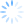Login/Register Math Worksheets, Activities, Games Helpline 099826-99306

# Grade 3 Math Worksheets: Printable worksheet for 3rd grade maths students

Presented below are 3 worksheets for Grade 3 Math students. You can download and print these worksheets for free and practice select topics including properties of addition, place value and even-odd numbers for class 3 Maths. These worksheets been designed by the expert team at BrainX Maths Program. They believe that for effective learning of math, 2 conditions need to be met.

• Maths must be Active. It is proven that not just grade 3 students but even adults retain up to 45 times more when they DO things as against just hear them
• Maths must be Fun: For kids to learn well, especially young students studying math in grade 3, they must enjoy it. Only when they have fun with Math, will they appreciate it and get better at it

Below, 3 worksheets for grade 3 math are presented. Each worksheet has a major focus and a minor focus depending upon the grade 3 math syllabus. Read on to discover more.

Math syllabus for grade 3 students now includes addition of 2 and 3 digit numbers. They can speed up their addition by up to 30% if they understand the properties of addition. The complete BrainX maths program for class 3 includes 18 worksheets on properties of addition. This includes associative property, commutative property, identity property and distributive property. Here we present "the temple", our intermediate level worksheet on addition properties. The objective is to match the left and right "flip flops".Worksheet focus  Grade for this worksheet: Class 3 Maths Major focus of this worksheet: Properties of addition Minor focus of this worksheet: Addition practice Click here to download more printable math worksheets for grade 3 . These worksheets are part of the Brainx program where printed worksheets are delivered to your home every month for the duration of the program. The program follows NCERT India and NCTM Singapore Maths curriculum for class 3 and address maths skills through applications, activities and games.

## 2nd worksheet for Grade 3 Math: Bonnie Tales for Place value

Place value and number systems form the key building blocks for any math skill and this is especially critical for grade 3 math students. In the complete BrainX program, class 3 students are trained in larger numbers (up to 5 digits), number to words, place value and order of magnitude through application and game based worksheets. Number system training takes up to 34 worksheets.

The objective of this worksheet is to join the dogs with the bones i.e. dog where the place value of underlined number is same as that on the bone. This worksheet is a genuine test of knowledge of place valueWorksheet focus  Grade for this worksheet: Class 3 Maths Major focus of this worksheet: Place value Minor focus of this worksheet: Concept of large numbers Click here to download more math worksheets for grade 3. These worksheets are aligned to NCTM Singapore and NCERT India curriculum guidelines for class 3 maths. The design of the worksheets ensures that students actively DO maths. So there is drawing, cutting, pasting, painting, solving and playing. All while practicing math for grade 3.

## 3rd worksheet for Grade 3 Math: the number pyramid for mixed skills

Ability to work through addition and subtraction in the same problem is the best indicator of knowledge and understanding. For grade 3 math students, we introduce number pyramid. These are challenging games with complex problem design. Over time, they are made more complex and students are asked to apply addition and subtraction simultaneously.

This is an advanced maths worksheet for 3rd grade students where they must complete the number pyramid. The number on the top is sum of the 2 numbers below it. The class 3 students must work their way up and down to complete this number pyramid.Worksheet focus  Grade for this worksheet: Class 3 Maths Major focus of this worksheet: Problem solving ability Minor focus of this worksheet: Addition and subtraction Download more printable math worksheets for grade 3 . Several more very innovative and propritory worksheets are included in the complete program. Students who enroll the complete program receive 20-25 printed worksheets at their home, every month. The worksheets move with the class curriculum and address the complete course for grade 3 math.  Thereby dramatically improving retention and understanding of math topics among students of 3rd class

These math worksheets for grade 3 are designed by BrainX Maths Program and presented here as samples. You can download more free sample worksheets from here. The free samples are updated on a timely manner and you keep coming back for more free worksheets.